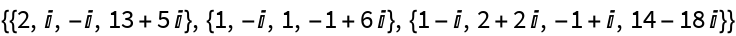#Function Repository Resource:

# ColumnSpaceBasis

Return a basis for the subspace spanned by the columns of a matrix

Contributed by: Dennis M Schneider
 ResourceFunction["ColumnSpaceBasis"][mat] returns a basis for the column space of mat.

## Details and Options

The basis returned is comprised of the pivot columns of the matrix.

## Examples

### Basic Examples

Compute the column space basis of a matrix:

 In:=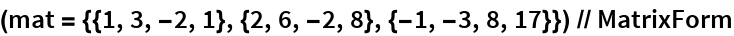Out=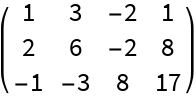The first and third columns of the matrix are pivot columns:

 In:=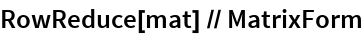Out=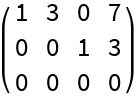The first and third columns of the matrix form a basis for the column space:

 In:=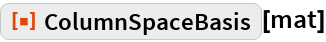Out=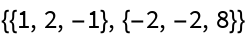Find a column space basis for a complex matrix:

 In:=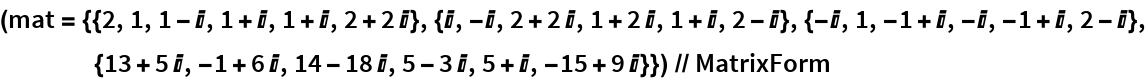Out=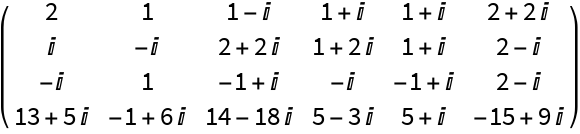The first three columns are pivot columns:

 In:=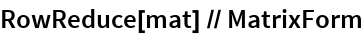Out=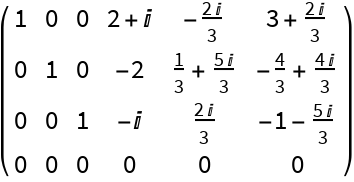The first three columns of the matrix form a basis for its column space:

 In:=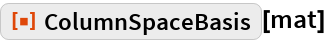Out=